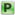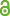Hauptmenü
• Autor
• Aistleitner, Christoph
• Berkes, István
• Seip, Kristian
• TitelGCD sums from Poisson integrals and systems of dilated functions
• Datei
• LicenceCC BY
• Zugriffsrechte• AbstractUpper bounds for GCD sums of the form $\sum_{k,{\ell}=1}^N\frac{(\gcd(n_k,n_{\ell}))^{2\alpha}}{(n_k n_{\ell})^\alpha}$ are proved, where $(n_k)_{1 \leq k \leq N}$ is any sequence of distinct positive integers and $0<\alpha \le 1$; the estimate for $\alpha=1/2$ solves in particular a problem of Dyer and Harman from 1986, and the estimates are optimal except possibly for $\alpha=1/2$. The method of proof is based on identifying the sum as a certain Poisson integral on a polydisc; as a byproduct, estimates for the largest eigenvalues of the associated GCD matrices are also found. The bounds for such GCD sums are used to establish a Carleson--Hunt-type inequality for systems of dilated functions of bounded variation or belonging to $\lip12$, a result that in turn settles two longstanding problems on the a.e.\ behavior of systems of dilated functions: the a.e. growth of sums of the form $\sum_{k=1}^N f(n_k x)$ and the a.e.\ convergence of $\sum_{k=1}^\infty c_k f(n_kx)$ when $f$ is 1-periodic and of bounded variation or in $\lip12$.